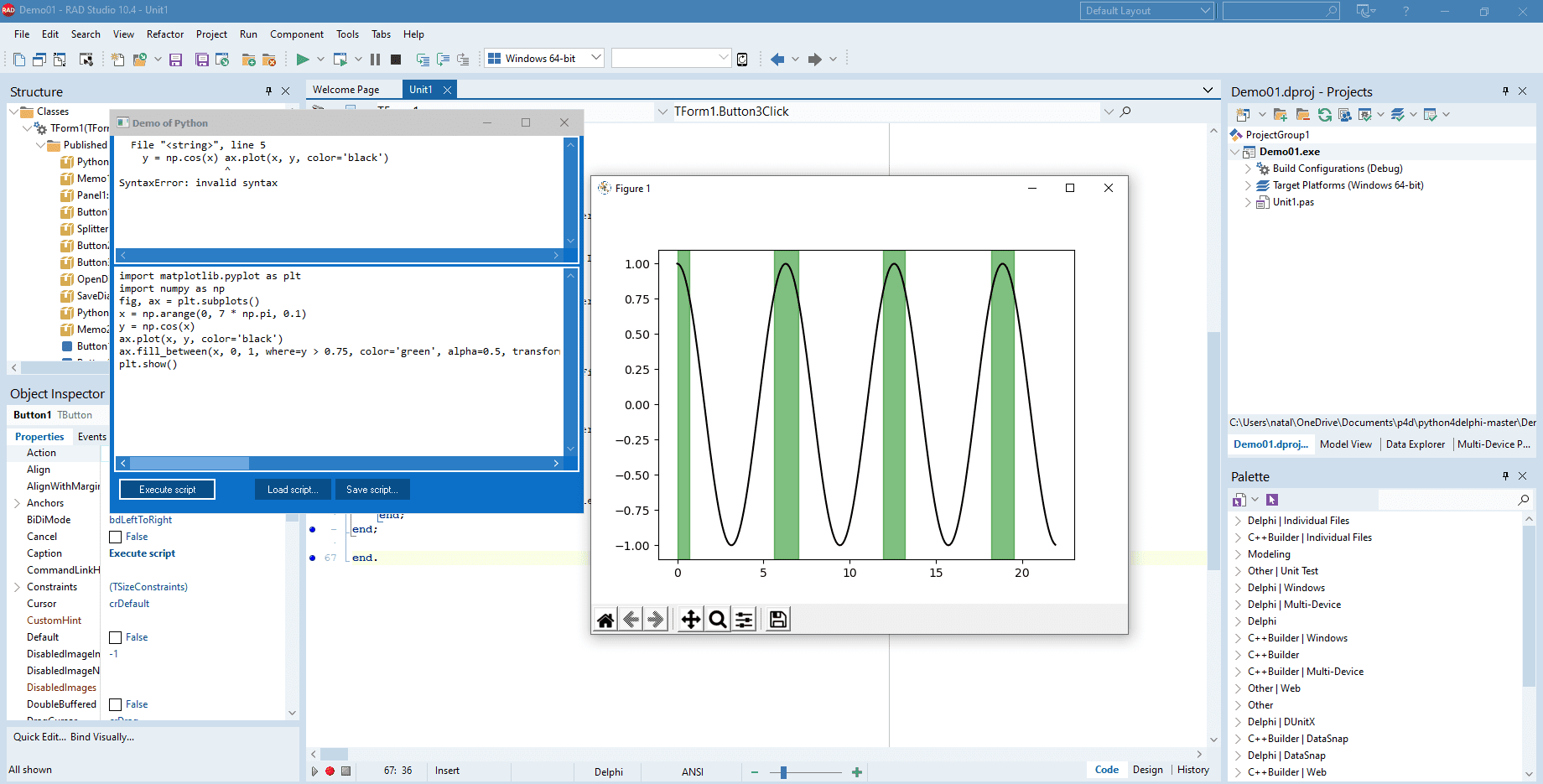# Learn To Build A Python GUI With The Matplotlib Library And A Delphi Windows GUI AppDrawing graphics programmatically is a very popular task these days. You can easily solve it using Matplotlib library with Python4Delphi (P4D). P4D is a free set of instruments that allows you to work with Python scripts, modules and types in Delphi. In this post, we will look at how to run Matplotlib library using Python for Delphi.

With Delphi and C++Builder and Python4Delphi, you can build Python GUI apps for Windows using various Python libraries. Open project Demo1 to run the Python script in Python for Delphi. Use Memo1 for Python script and Memo2 for results. Click Execute button for running the script. Download Demo1 source from GitHub. If you run into a floating point division error when executing the code run MaskFPUExceptions(True); before you call ExecStrings.

Python Matplotlib library provides various tools for working with 2D graphics. With this library, you can create graphics, customize legends, style sheets, color schemes, and manipulate images. There are examples of code from the Matplotlib below.

### Draw a plot

You can draw very simple plots with Mathplotlib. Or, if you want, you can change the shape and color of the points in the graphic in different ways. In the following example, we will draw the green triangular points using the argument ‘g^’  in function plot().

### Stacked bar chart

In this example, we show how to draw a stacked bar plot. We will use the function bar() twice. We need to pass to this function such parameters as labels, values of various categories that need to be displayed, names of labels. Finally, we will set such graphic parameters as title, y-label.

### Draw curves and fill the area

In this example, we use the function plot() again to build a graphic of cos(x). Then we fill the area in green color between two curves using function fill_between(). Pass parameters x, y1 and y2 to determine the curve and exclude some horizontal regions from being filled using parameter where.We got acquainted with some of the Matplotlib library’s features. Go here, download this library and draw your beautiful graphs. Download Python4Delphi and build Python GUIs for Windows using Delphi easily.

### Watch the Python GUI Apps Con 2023 sessions today!